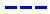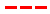Disneyland attraction wait times for November 12, 2023.

•Average Wait Time We PredictedAverage Wait Time We Saw
•Average Wait Time We PredictedAverage Wait Time We Saw
•Average Wait Time We PredictedAverage Wait Time We Saw
•Average Wait Time We PredictedAverage Wait Time We Saw
•Average Wait Time We PredictedAverage Wait Time We Saw
•Average Wait Time We PredictedAverage Wait Time We Saw
•Average Wait Time We PredictedAverage Wait Time We Saw
•Average Wait Time We PredictedAverage Wait Time We Saw
•Average Wait Time We PredictedAverage Wait Time We Saw
•Average Wait Time We PredictedAverage Wait Time We Saw
•Average Wait Time We PredictedAverage Wait Time We Saw
•Average Wait Time We PredictedAverage Wait Time We Saw
•Average Wait Time We PredictedAverage Wait Time We Saw
•Average Wait Time We PredictedAverage Wait Time We Saw
•Average Wait Time We PredictedAverage Wait Time We Saw
•Average Wait Time We PredictedAverage Wait Time We Saw
•Average Wait Time We PredictedAverage Wait Time We Saw
•Average Wait Time We PredictedAverage Wait Time We Saw
•Average Wait Time We PredictedAverage Wait Time We Saw
•Average Wait Time We PredictedAverage Wait Time We Saw
•Average Wait Time We PredictedAverage Wait Time We Saw
•Average Wait Time We PredictedAverage Wait Time We Saw
•Average Wait Time We PredictedAverage Wait Time We Saw
•Average Wait Time We PredictedAverage Wait Time We Saw
•Average Wait Time We PredictedAverage Wait Time We Saw
•Average Wait Time We PredictedAverage Wait Time We Saw
•Average Wait Time We PredictedAverage Wait Time We Saw
•Average Wait Time We PredictedAverage Wait Time We Saw
•Average Wait Time We PredictedAverage Wait Time We Saw
•Average Wait Time We PredictedAverage Wait Time We Saw
•Average Wait Time We PredictedAverage Wait Time We Saw
•Average Wait Time We PredictedAverage Wait Time We Saw
•Average Wait Time We PredictedAverage Wait Time We Saw
•Average Wait Time We PredictedAverage Wait Time We Saw
•Average Wait Time We PredictedAverage Wait Time We Saw
•Average Wait Time We PredictedAverage Wait Time We Saw